Computational Biology and Bioinformatics
Volume 4, Issue 4, August 2016, Pages: 27-36

Review Article

Detection of Abnormality in Electrocardiogram (ECG) Signals Based on Katz’s and Higuchi’s Method Under Fractal Dimensions

Md. Mashiur Rahman1, A. H. M. Zadidul Karim2, Abdullah Al Mahmud2, *, Salma Nazia Rahman2

1Department of EEE, Ahsanullah University of Science and Technology, Dhaka, Bangladesh

2Department of EEE, University of Asia Pacific, Dhaka, Bangladesh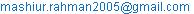(Md. M. Rahman)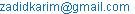(A. H. M Z. Karim)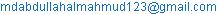(A. A. Mahmud)(S. N. Rahman)

*Corresponding author

Md. Mashiur Rahman, A. H. M. Zadidul Karim, Abdullah Al Mahmud, Salma Nazia Rahman. Detection of Abnormality in Electrocardiogram (ECG) Signals Based on Katz’s and Higuchi’s Method Under Fractal Dimensions. Computational Biology and Bioinformatics. Vol. 4, No. 4, 2016, pp. 27-36. doi: 10.11648/j.cbb.20160404.11

Received: July 28, 2016; Accepted: August 9, 2016; Published: September 5, 2016

Abstract: Analysis process of electrocardiogram (ECG) is a major research interests in bio-medical signal processing. The reasons of this interest is the growth of cardiac health care activities all over the world and the rapid progress in digital computer technology which play an essential role to the detection of diseases at various stages from bio medical signals. The assessment process of diagnostic results for these bio medical signals heavily depends upon quantity, accuracy and speed. Computer based analysis is very useful in clinical therapy. In this Paper a method of analysis (ECG) signals using fractal features have been proposed and practical experiments have done to show that this method provides a good electronic diagnosis pattern for cardiac abnormality because it has been used by some specialist doctors to diagnose various types of diseases with accuracy. By the fact that ECG signals show a fractal patterns, it has been tried to find out a comparison between Katz’s and Higuchi’s method under fractal dimension (FD) of the ECG time series in a feature extraction phase. All ECG signals have been acquired from the Massachusetts Institute of Technology (MITBIH) arrhythmia database. The obtained results confirm the superiority of the Katz’s and Higuchi’s method to identify cardiac abnormality as compared to traditional one which is analyses of ECG signals based on morphology features and three ECG temporal features.(i.e. the QRS complex duration, the RR interval and the RR interval averaged over the ten last beats).

Keywords: ECG, Katz’s Method, Higuchi’s Method, Fractal Features, Heart

Contents

1. Introduction

1.1. Heart and It’s Relation with ECG

In humans, the heart is roughly the size of a large fist and weighs between about 10 to 12 ounces (280 to 340 grams) in men and 8 to 10 ounces (230 to 280 grams) in women, according to Henry Gray's "Anatomy of the Human Body [1-2]". The physiology of the heart basically comes down to "structure, electricity and plumbing," Phillips told Live Science . Human heart consists of two parts. One is left part and another one is right. The right atrium and right ventricle together make up the "right heart," and the left atrium and left ventricle make up the "left heart." A wall of muscle called the septum separates the two sides of the heart. A double-walled sac called the pericardium encases the heart, which serves to protect the heart and anchor it inside the chest. Between the outer layer, the parietal pericardium, and the inner layer, the serous pericardium, runs pericardial fluid, which lubricates the heart during contractions and movements of the lungs and diaphragm. The heart's outer wall consists of three layers. The outermost wall layer, or epicardium, is the inner wall of the pericardium. The middle layer, or myocardium, contains the muscle that contracts. The inner layer, or endocardium, is the lining that contacts the blood. The tricuspid valve and the mitral valve make up the atrioventricular (AV) valves, which connect the atria and the ventricles. The pulmonary semi-lunar valve separates the right ventricle from. The pulmonary artery, and the aortic valve separates the left ventricle from the aorta. The heartstrings, or chordae tendinae, anchor the valves to heart muscles. The sinoatrial node produces the electrical pulses that drive heart contractions.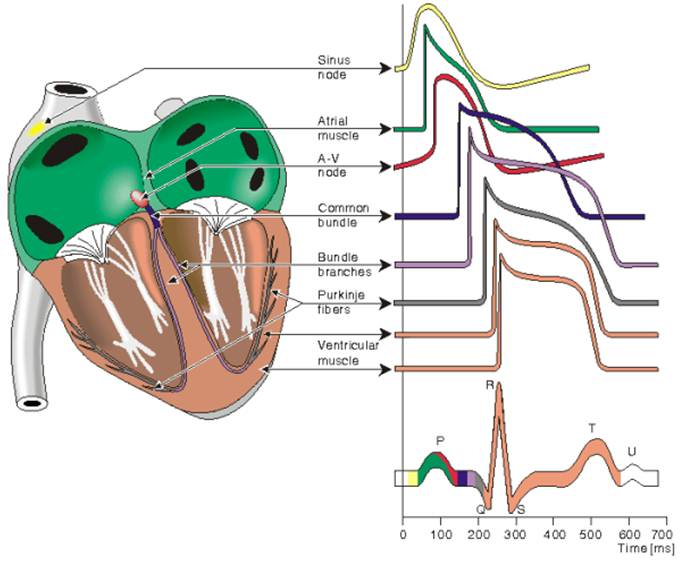Figure 1. Propagation of the Action Potential through heart .

In a normal ECG, there are three distinct waves. Together these waves represent one heartbeat. The waves give a signal what's happening in the heart at a certain time. The first wave is called the P wave. It's a relatively small wave. It represents the depolarization of the atria. Depolarization is defined as the change in the cell's membrane potential to a more positive state. It's this change that generates the electrical impulse that starts the heart's contraction. So it can associate with the P wave of an ECG with the contraction of the atria. In the graph of the ECG, a small dip is followed by a large spike and another dip. This series is usually considered together, and it's called the QRS wave. It is seen that this puts the waves in alphabetical order. The QRS wave is sometimes called the QRS complex, and it represents the depolarization of the ventricles. This quickly leads to the contraction of the ventricles and ejection of blood out of the heart and into the large arteries exiting the heart. So the QRS wave is associated with the contraction of the ventricles, so QRS wave is associated with the beginning of systole, because systole is the phase of the cardiac cycle where the ventricles contract [6-10].

1.2. Fractal Features

Two of the most important properties of fractals are self-similarity and non-integer dimension . Fractal Dimension can be demonstrated by first defining a fractal set as: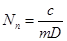(1)

Where Nn is the number of fragments with the linear dimension defined as m, C is some constant, and D defines the fractal dimension. If this equation is rearranged with simple algebra, the outcome is: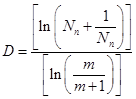(2)

Given a line of unit length, it can be divided in varying ways and do different things with each segment. For the figure 2, if the segment is divided into two parts, making r1 =0.5, where r1 is the length of the division . One of the parts is kept and the other is disposed of, so N1 =1. If the remaining segment is divided into two parts and again only keep one of the fragments, then r2 = 1/4 and N2 =1. If this process is repeated (iterated), D turns out to be zero, which gives the equivalent to the Euclidean point. Regardless of the number of iterations, at order n, N2=1. Hence, D will always be zero. This way of thinking makes sense because if a line of segment is taken and continually divide it into two by keeping only one of the pieces, the length of the line segment will approach zero as the order approaches infinity.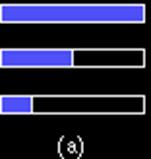Figure 2. Demonstration of fractal dimensions with Euclidean line segments.

2. Methodology

For certain objects which will have to deal through human life, such as squares, lines, and cubes, it is easy to assign a dimension. It is felt that a square has two dimensions, a line has one dimension, and a cube has three dimensions. By retrospection at figure 2 it is seen that it has been divided into pieces that when zoomed in on n times, reappeared to the starting figure. Because of this dividing the ln(number of divisions) by the natural logarithm of the magnification factor. The resulting formula gives the dimension, represented by D .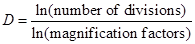(3)

For a line: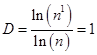(4)

The above example is very easy because the magnification factor is always n. But for fractals, magnification factor will be a constant which varies for each fractal.

Herein, fractal complexity of signal is characterized in real-time by computing its FD using each of Katz’s method and Higuchi’s method.

2.1. Katz’s Method

The FD of a signal curve based on Katz’s method  can be defined as:

FD = log (L)/log (d)

Where, L is the total signal curve length or sum of distance between successive points, and d is the diameter estimation of the distance between the first data point and the data which gives the farthest distance.

d and L, are respectively, can be expressed mathematically as below: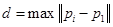(5)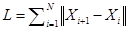(6)

Normalizing distances in (1) by the average distance between successive points, say a, gives: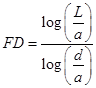(7)

Defining n as the number of steps in the signal curve less than the number of points N, then n = L/a. Substituting n in (2), FD according to Katz’s approach is expressed as: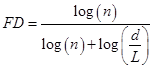(8)

2.2. Higuchi’s Method

Higuchi proposed an efficient algorithm to calculate the FD directly from time series . Assume a one dimensional time series X= {X(1), X(2), X(3), …, X(N)} where, N is the total number of samples, in this case the series X would be the successive values of ECG signal. The Higuchi’s algorithm constructs k new time series as: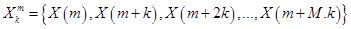(9)

where k and m are integers, represent time interval between points and initial time value respectively,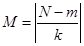(10)

For each new time series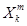constructed the length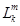is computed as: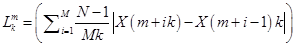(11)

where,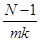is a normalization factor for the curve length of.

The length of the series L(k) for the time interval k is computed as the mean of the k values, for m = 1, 2,. .., k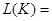1/K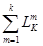(12)

If L (k) is proportional to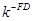, then the curve describing the shape of ECG time series is fractal-like with the dimension FD. In this case, if ln(L(k)) is plotted against ln(k), k = 1, 2, 3,. ..,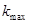, the points fall on a straight line with a slope equal to FD. The fractal dimension of ECG signal is calculated via above method while applying adaptive and fixed windowing method.

3. Analysis Procedure and Simulation Results in Brief

3.1. Dataset for Analysis Procedure

In this Paper a fractal dimension (FD) for 31 dataset of ECG signals has been determined in time domain then ranges of FD is established for a healthy person and persons with various heart diseases. The sample of ECG signals for the present study is obtained from MIT/BIH database via Physionet website . The MIT-BIH database contains both normal and abnormal types of ECG signals. In this study, the considered beats are referred to the following classes (i.e Normal Sinus Rhythm (N), Premature Ventricular Contraction (PVC), Atrial Premature (AP), Right Bundle-Branch Block (RBBB), and Left Bundle-Branch Block (LBBB)). The beats were selected from the recording of 31 persons which correspond to the following files: 17052m, 16420m, 19088m, 19093m, 16265m, 16483m, 16273m, 16549m, 16539m, 16795m, 17453m, 18177m, 18184m, 19090m, 19830m, 16786m, 16277m, 16792m and 16272m for Normal Sinus Rhythm (N). 200m, 208m, and 215m for Premature Ventricular Contraction (PVC). 100m, 209m, and 223m for Atrial Premature (AP). 124m, 231m and 232m for Right Bundle-Branch Block (RBBB). 214m, 109m, and 217m for Left Bundle-Branch Block (LBBB). The properties of these signals are described in Table 1. Figure 3 to Figure 7 show the plot of two dataset for each types of signal. It is mentioned that all data that has been used in this paper are applicable for male patients. For this reason English small letter "m" is written with every data set of patient.

Table 1. Description of the used dataset.

 Signal Type No of Samples Sampling frequency (Hz) Sample Intervals (Sec) Ventricular Premature Arrhythmia (VPA) 3600 360 .6500000 Artial Premature (AP) 3600 360 .6500000 Right bundle-branch block (RBBB) 3600 360 .6500000 Left bundle branch block (LBBB) 3600 360 .6500000 Normal sinus rhythm (NSR) 1280 128 .6500000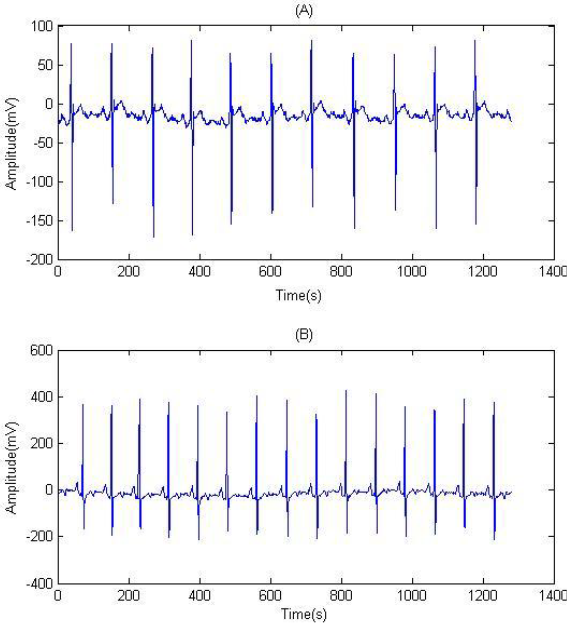Figure 3. (A), (B) ECG signals of Normal Rhythm.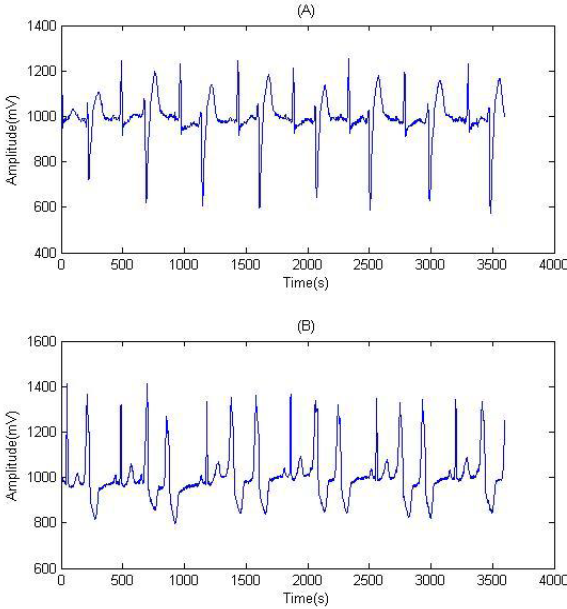Figure 4. (A), (B) ECG signals of a Premature Ventricular Arrhythmia.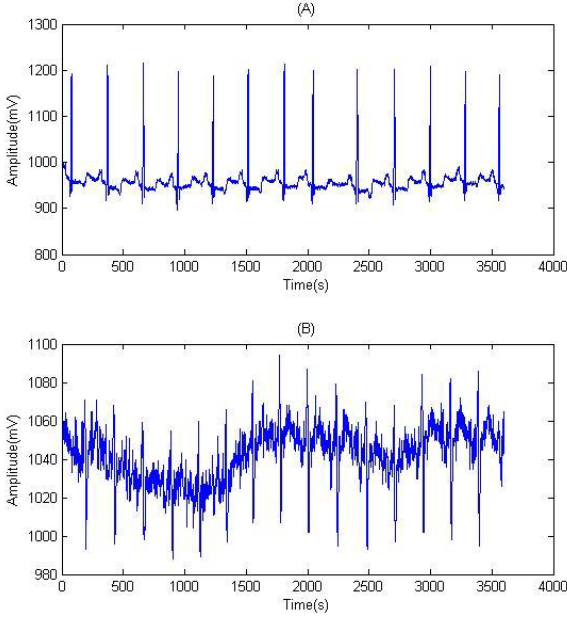Figure 5. (A), (B) ECG signals of a Atrial Premature Arrhythmia.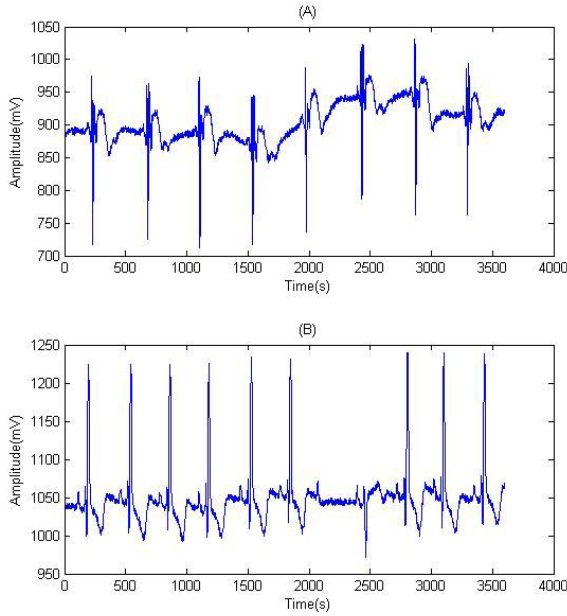Figure 6. (A), (B) ECG signals of Right Bundle-Branch Block Arrhythmia.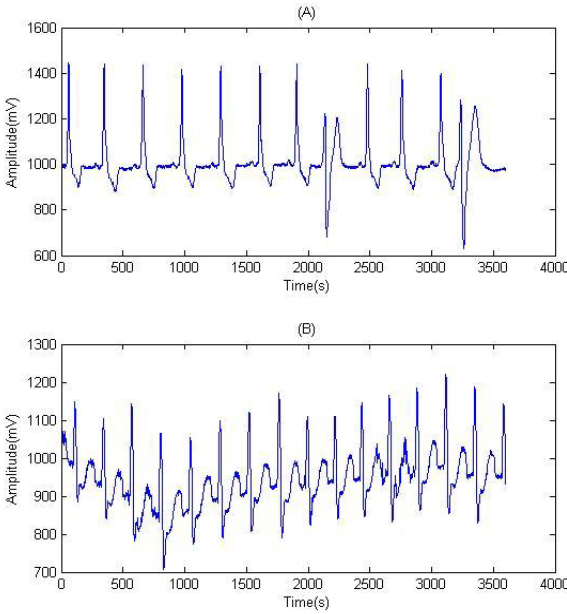Figure 7. (A), (B) ECG signals of Left Bundle-Branch Block Arrhythmia.

3.2. Simulation Results of Fractal Features Extraction

In order to feed the classification process, fractal features of the ECG signals will have to extract. To do so 31 dataset have been utilized which are composed of ECG signals recorded from healthy subjects and patients with heart arrhythmia. The experiments have been performed using Matlab 7 on ECG datasets from the MITBIH arrhythmia database . The FD feature from each class of ECG time series signal has extracted. Table 2 shows the results obtained for estimating FD from the Normal heart rhythm signals, which prove that the healthy heart is the fractal heart; since the value of FD lies between 1 and 2. Table 3-6 show the results obtained for the estimation of FD from the pathological signals, and table 7, 8 show the intervals (lower bound and upper bound) of the estimated FD for each specific disease corresponding to each estimation method. By comparing these estimated FD intervals shown in table 7 and table 8. It is clear that both Katz’s and Higuchi’s method can distinguish obviously between healthy and non-healthy persons by putting each of them in distinct FD range. On the other hand, Table 9 shows the average of FD values for each of ECG signal type along with the estimated methods that are used. The Values of other heart arrhythmia (i.e Left Bundle Branch Block, Right Bundle Branch Block, Atrial Premature and Ventricular Premature Arrhythmia) are lower and equal to 0.742733, 0.438833, 0.249733, and 0.082267 respectively. There is a decrease in the average of FD value, this decrease in the FD value indicates a decrease in the heterogeneity of the cardiac recording . Meanwhile, if the average FD value of Normal heart rhythms are compared with Abnormal heart rhythms that are obtained by each of the time domain methods (i.e. Katz’s and Higuchi’s method), it is clear that both katz’s and Higuchi’s can accurately distinguish between normal and abnormal condition.

Table 2. The Estimated FD Values for Normal Sinus Rhythm Signals.

 Data Sets FD estimation methods Katz Higuchi's 1. 17052m 2.1743 1.6051 2. 16420m 2.1372 1.5768 3. 19088m 1.7247 1.1140 4. 19093m 1.6402 1.0492 5. 16265m 2.4422 1.7299 6. 16483m 2.1783 1.3252 7. 16273m 2.2544 1.4789 8. 16549m 2.1744 1.2713 9. 16539m 2.0761 1.5325 10. 16795m 2.0527 1.3285 11. 17453m 2.1010 1.4149 12. 18177m 2.1240 1.3793 13. 18184m 2.2229 1.4506 14. 19090m 1.7677 1.1015 15. 19830m 1.6790 1.0295 16. 16786m 1.9652 1.5126 17. 16277m 1.8652 1.3061 18. 16792m 2.3240 1.5122 19. 16272m 1.9763 1.5072Figure 8. Illustration of Katz’s and Higuchi’s curve for Normal Sinus Rhythm Signals.

Table 3. The Estimated FD Values for Ventricular Premature Arrhythmia Signals.

 Dataset (3600 beats) FD estimation methods Katz Higuchi's 1. 200m 1.6248 1.1312 2. 208m 1.6607 1.1616 3. 215m 1.8216 1.4922Figure 9. Illustration of Katz’s and Higuchi’s curve for Ventricular Premature Arrhythmia Signals.

Table 4. The Estimated FD Values for Atrial Premature Arrhythmia Signals.

 Dataset (3600 beats) FD estimation methods Katz Higuchi's 1. 100m 1.8583 1.3014 2. 209m 2.2321 1.4429 3. 223m 1.6217 1.1095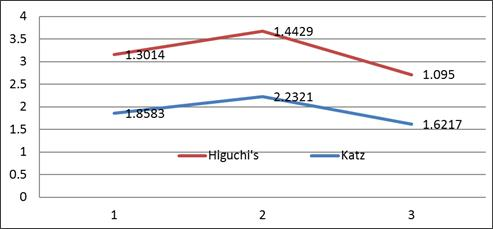Figure 10. Illustration of Katz’s and Higuchi’s curve for Atrial Premature Arrhythmia Signals.

Table 5. The Estimated FD Values for Right Bundle Branch Block Arrhythmia Signals.

 Dataset (3600 beats) FD estimation methods Katz Higuchi's 1. 124m 1.8544 1.2683 2. 231m 1.7198 1.1971 3. 232m 1.8760 1.2378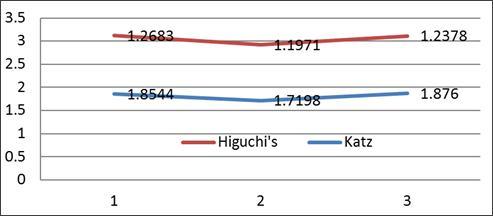Figure 11. Illustration of Katz’s and Higuchi’s curve for Right Bundle Branch Block Arrhythmia Signals.

Table 6. The Estimated FD Values for Left Bundle Branch Block Arrhythmia Signals.

 Dataset (3600 beats) FD estimation methods Katz Higuchi's 1. 214m 1.6661 1.1670 2. 109m 1.7174 1.1585 3. 217m 1.6644 1.1259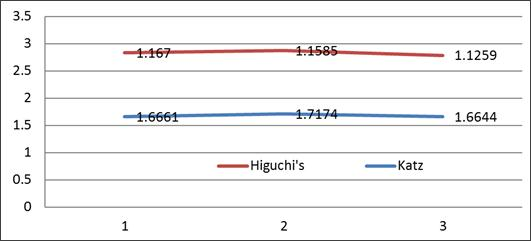Figure 12. Illustration of Katz’s and Higuchi’s curve for Left Bundle Branch Block Arrhythmia Signals.

Table 7. Ranges of FD Values for Sample ECG Signal Using Katzs Method.

 Signal Type Range Percentage of accuracy Ventricular Premature Arrhythmia (VPA) 1.6248-1.8216 19.68 Atrial Premature (AP) 1.6217-2.2321 61.04 Right Bundle Branch Block (RBBB) 1.7198-1.8760 15.62 Left Bundle Branch Block (LBBB) 1.6644-1.7174 5.31 Normal Sinus Rhythm (NSR) 1.6402-2.4422 80.2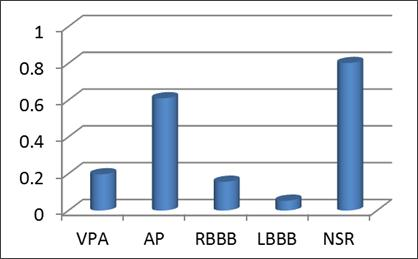Figure 13. Illustration of ranges for Sample ECG Signal Using Katz’s Method.

Table 8. Ranges of FD Values for Sample ECG Signal Using Higuchis Method.

 Signal Type Range Percentage of accuracy Ventricular Premature Arrhythmia (VPA) 1.1312-1.4922 36 Atrial Premature(AP) 1.1095-1.4429 33.32 Right Bundle Branch Block(RBBB) 1.1971-1.2683 7.12 Left Bundle Branch Block(LBBB) 1.1259-1.1670 4.11 Normal Sinus Rhythm(NSR) 1.0295-1.7299 71.04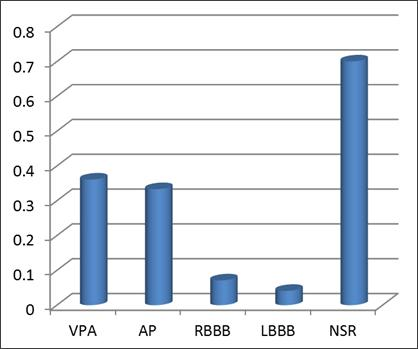Figure 14. Illustration of ranges for FD Values of Sample ECG Signal Using Higuchi’s Method.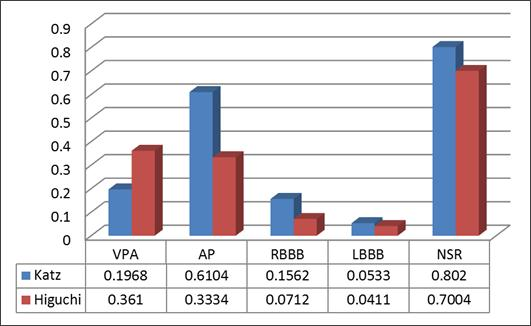Figure 15. Comparative Illustration of ranges for FD Values of Sample ECG Signal Using Katz’s and Higuchi’s Method.

Table 9. Average of the Estimated FD Values.

 Signal Type Katz Higuchi's Ventricular Premature Arrhythmia (VPA) 1.702367 1.261667 Atrial Premature (AP) 1.904033 1.284600 Right Bundle Branch Block (RBBB) 1.816733 1.234400 Left Bundle Branch Block (LBBB) 1.682633 1.150467 Normal Sinus Rhythm (NSR) 2.046305 1.380279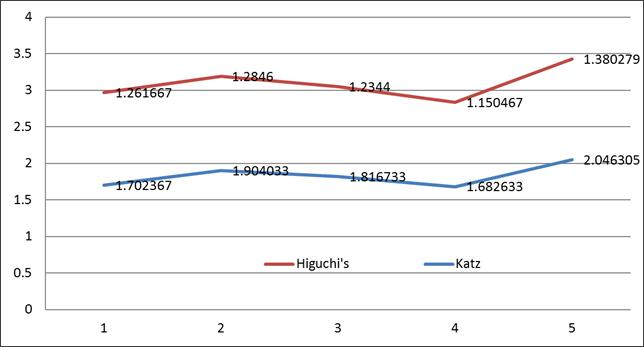Figure 16. Illustration of Katz’s and Higuchi’s curve for average estimated FD values.

4. Conclusion

In this paper katz’s and Higuchi’s method under FD dimension have been studied. Normality (i.e. Normal sinus rhythm) and abnormality (i.e. Ventricular premature arrhythmia, Right bundle branch block, Left bundle branch block, Atrial Premature) have been detected by both method. From the illustration of table 7 it is seen that in katz method the percentage of accuracy to detect Normal sinus rhythm is 80%, atrial premature is 61.04%, Right bundle block is 15.62%, Left bundle block is 5.31%, Ventricular premature arrhythmia is 19.68%. Again from the illustration of table 8 it is seen that in Higuchi’s method the percentage of accuracy to detect normal sinus rhythm is 71.04%, atrial premature is 33.34%, Right bundle branch block is 7.12%, Left bundle branch block is 4.11%, Ventricular premature arrhythmia is 36%. Comparing this two method it can be said that Katz’s method is more efficient to detect normal sinus rhythm and atrial premature by comparison with Higuchi’s method. It is also observed that Higuchi’s method is more efficient to detect atrial premature by comparison with Katz method.

References

1. http://www.bartleby.com/107/138.html
2. http://www.bartleby.com/107/136.html
3. http://www.vhlab.umn.edu/atlas/physiology-tutorial
4. http://www.livescience.com/34655-human-heart.html
5. http://www.j-circ.or.jp/english/sessions/reports/64th-ss/nerbonne-l1.htm
7. "ECG-simplified. Aswini Kumar M. D.". LifeHugger. Retrieved 11 February 2010.
8. Walraven, G. (2011). Basic arrhythmias (7th ed.), pp. 1–11.
9. Takemi Program in International Health Dr. Taro Takemi
10. Mark, Jonathan B. (1998). Atlas of cardiovascular monitoring. New York: Churchill Livingstone. ISBN 0-443-08891-8.
11. Falconer, Kenneth (2003). Fractal Geometry. New York: Wiley. p. 308. ISBN 978-0-470-84862-3.
12. http://library.thinkquest.org/3493/ frames/- fractal.html.
13. J. M. Blackledge, (2006), " Digital Signal Processing: Mathematical and Computation Methods: Software Development and Applications", 2nd Edition, London: Horwood Publishing Limited.
14. F. Melgani and L. Bruzzone, (Aug. 2004), "Classification of Hyperspectral Remote Sensing Images with Support Vector Machine", IEEE Trans. Geosci, Remote Sens, vol. 42, no. 8: pp. 1778–1790.
15. MIT-BIH Arrhythmia Database from PhysioBank- Physiologic Signal Archives for Biomedical Research. Retrieved 10 March, 2013, from http://www.physionet.org/ -physiobank/database.
16. Accardo A., Affinito M., Carrozzi M, Bouquet F, (1997) " Use of the Fractal Dimension for the Analysis of Electroencephalographic Time Series", Biol Cyber, 77: 339-350.

 Contents 1. 1.1. 1.2. 2. 2.1. 2.2. 3. 3.1. 3.2. 4.
Article ToolsAbstractPDF(3209K)